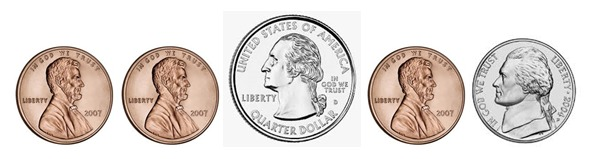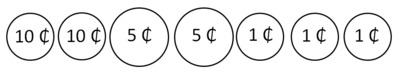# Jamir's Penny Jar

Alignments to Content Standards: 2.MD.C.8 2.NBT.B.5

Jamir has collected some pennies in a jar. Recently, he added coins other than pennies to his jar. Jamir reached his hand into the jar and pulled out this combination:1. Jamir wants to count the total value of these coins. What coin do you suggest he start with? Why would Jamir want to start counting with this coin?
2. What is the total value of these coins? Write a number sentence that represents the total value of the coins.
3. Jamir reached into the jar again and was surprised to pull out a different combination of coins with the same total value as before. Draw a collection of coins that Jamir could have pulled from the jar. Write a number sentence that represents the total value of the coins.

## IM Commentary

The purpose of this task is to help students articulate their addition strategies as in 2.NBT.5 and would be most appropriately used once students have a solid understanding of coin values. It also provides a context where it makes sense to “skip count by 5s and 10s” (as described in 2.NBT.2) for the combinations that involve more than one nickel or dime. The context provides a link to 2.MD.8. This task would be best used in an instructional setting especially since the language is somewhat complex and the teacher might need to help students decode the task statement.

The Standards for Mathematical Practice focus on the nature of the learning experiences by attending to the thinking processes and habits of mind that students need to develop in order to attain a deep and flexible understanding of mathematics. Certain tasks lend themselves to the demonstration of specific practices by students. The practices that are observable during exploration of a task depend on how instruction unfolds in the classroom. While it is possible that tasks may be connected to several practices, only one practice connection will be discussed in depth. Possible secondary practice connections may be discussed but not in the same degree of detail.

This particular task supports the demonstration of Mathematical Practice Standard 4, Model with mathematics. Students apply the mathematics they know to solve problems arising in everyday life. For this problem, young children might skip count, apply their addition strategies, or write an addition sentence to describe the situation. This problem is complex in that students may need assistance decoding the language and there are multiple solutions for Part C. Students identify the mathematical elements of the situation and decide what solution pathway is best for them to follow. Because there are multiple solutions to Part C, students have ample opportunities to explain their solutions to each other and critique each other’s reasoning as in Mathematical Practice Standard 3.

## Solution

1. Jamir should start with a quarter because that has the largest value of all the coins. It is easiest to count up starting with the largest value and adding lesser values.

2. The total value of the coins is 33¢. One way to write the number sentence that would reflect the strategy of starting with the largest value and adding on the next largest values and so on would be

$$25 + 5 + 1+ 1 + 1 = 33$$

although students can write the addends in any order.

1. Note that only one picture is shown but students should draw a picture for the combination they chose. Students should be encouraged to write the cent symbol on their pictures. Possible answers:
• 1 quarter and 8 pennies

$$25 + 1 + 1+ 1 + 1 + 1 + 1+ 1 + 1= 33$$

• 3 dimes and 3 pennies

$$10 + 10 + + 10 +1+ 1 + 1 = 33$$

• 2 dimes, 2 nickels, and 3 pennies$$10 + 10 + 5 +5 + 1+ 1 + 1 = 33$$

• 2 dimes, 1 nickel, and 8 pennies

$$10 + 10 + 5 + 1 + 1+ 1 + 1 + 1 + 1+ 1 + 1 = 33$$

• 1 dime, 4 nickels, and 3 pennies

$$10 + 5 + 5 + 5 + 5 + 1+ 1 + 1 = 33$$

• 1 dime, 3 nickels, and 8 pennies

$$10 + 5 + 5 + 5 + 1 + 1+ 1 + 1 + 1 + 1+ 1 + 1 = 33$$

• 1 dime, 2 nickels, and 13 pennies

$$10 + 5 + 5 + 1 + 1+ 1 + 1 + 1 + 1 + 1+ 1 + 1 + 1 + 1+ 1 + 1 = 33$$

• 1 dime, 1 nickel, and 18 pennies

$$10 + 5 + 1 + 1+ 1 + 1 + 1 + 1 + 1+ 1 + 1 + 1 + 1 + 1+ 1 + 1 + 1 + 1+ 1 + 1 = 33$$

• 1 dime and 23 pennies
\begin{align} 10 &+ 1 + 1 + 1 + 1 + 1 + 1 + 1+ 1 + 1 + 1 + 1 + 1+ 1 + 1 + 1 + 1 + 1+ 1 \\ &+ 1 + 1 + 1+ 1 + 1 = 33 \end{align}
• 6 nickels and 3 pennies

$$5 + 5 + 5 + 5 + 5 + 5 + 1+ 1 + 1 = 33$$

• 5 nickels and 8 pennies

$$5 + 5 + 5 + 5 + 5 + 5 + 1+ 1 + 1 + 1 + 1 + 1 + 1 + 1 = 33$$

• 4 nickels and 13 pennies

$$5 + 5 + 5 + 5 + 1 + 1+ 1 + 1 + 1 + 1 + 1+ 1 + 1 + 1 + 1+ 1 + 1 = 33$$

• 3 nickels and 18 pennies

$$5 + 5 + 5 + 1 + 1+ 1 + 1 + 1 + 1 + 1+ 1 + 1 + 1 + 1 + 1+ 1 + 1 + 1 + 1+ 1 + 1 = 33$$

• 2 nickels and 23 pennies
\begin{align} 5 + 5 & + 1 + 1 + 1 + 1 + 1 + 1 + 1+ 1 + 1 + 1 + 1 + 1+ 1 + 1 + 1 + 1 + 1+ 1 \\ & + 1 + 1 + 1 + 1 + 1 = 33 \end{align}
• 1 nickel and 28 pennies
\begin{align} 5 & + 1 + 1 + 1 + 1 + 1 + 1 + 1 + 1 + 1 + 1 + 1 + 1+ 1 + 1 + 1 + 1 + 1+ 1 \\ & + 1 + 1 + 1 + 1 + 1 + 1 + 1 + 1 + 1 + 1 = 33 \end{align}
• 33 pennies
\begin{align} 1 & + 1 + 1 + 1 + 1 + 1 + 1 + 1 + 1 + 1 + 1 + 1 + 1 + 1 + 1 + 1 + 1 + 1 \\ & + 1 + 1 + 1 + 1 + 1 + 1 + 1 + 1 + 1 + 1 + 1 + 1 + 1 + 1 + 1 = 33 \end{align}